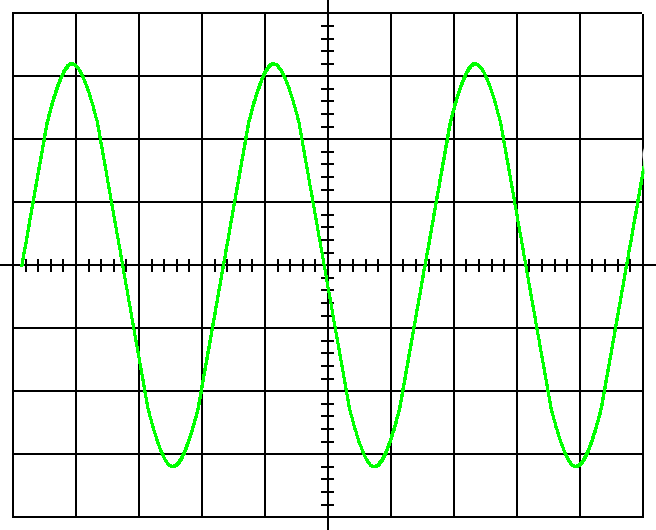# Oscilloscope Pre-lab Question

## Homework Statement

In the oscilloscope display above, the vertical sensitivity is 5mV/cm, and the horizontal sensitivity is 0.5μs/cm.
A) find the amplitude of the sinusoidal voltage
B) find the frequency of the sinusoidal voltagehttp://imgur.com/fnkXliz

## Homework Equations

im not sure which equations to use for this question, if any are needed.

## The Attempt at a Solution

I know you have to convert the units in this question im assuming. It also says in the question that each block is 1cm by 1 cm, and the top of the amplitude is about 3.2 cm, but that isn't the correct answer.

DEvens
Gold Member
Ask yourself, what does it mean when it says 5 mV/cm? And so, what does it mean if you have a wave 3.2 cm tall on the screen?

Ask yourself, what does it mean when it says 0.5μs/cm? And how long (in cm) is one wave of the signal on the screen? And so, what does that mean?

i think it means (and correct me if im wrong) that there is 5 megavolts (5000000 volts) in every centimeter on the y-axis. So if the wave is 3.2 cm tall would you multiple 5mV by 3.2 to get the amplitude???

berkeman
Mentor
i think it means (and correct me if im wrong) that there is 5 megavolts (5000000 volts) in every centimeter on the y-axis. So if the wave is 3.2 cm tall would you multiple 5mV by 3.2 to get the amplitude???

First of all, mV is milliVolts, not MegaVolts... :-)

Next, I see the vertical amplitude as a little over 6 divisions tall. So the peak-to-peak amplitude will be a bit over 6 * 5mVpp. It's hard to tell if the question is asking for the peak or peak-to-peak "amplitude". Can you clarify that?

And on the horizontal axis, how many divisions between the positive peaks of the waveform (or alternately use the positive-going zero crossings to find the waveform's period)

ehild
Homework Helper
http://imgur.com/fnkXliz
this is the graph posted in the question aswell

Better to show the picture:#### Attachments

Okay so i got the first question right, it was asking for the peak not the peak to peak. So for the second part about finding the frequency, I counted roughly 3.2 blocks as the period, so the frequency is just 1/period right? im not sure what to put in for units though, i thought frequency was measured in Hz, but when i try to put the answer in online it says the units are wrong... any help?

BvU